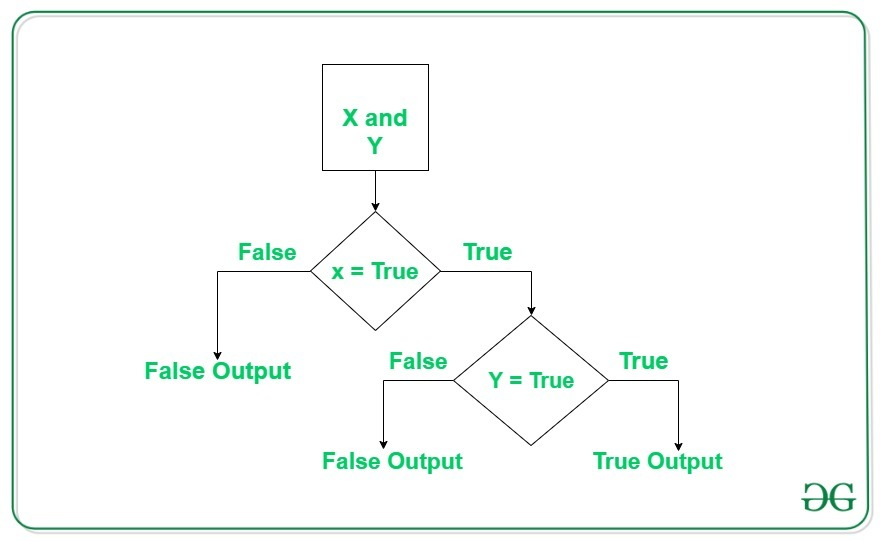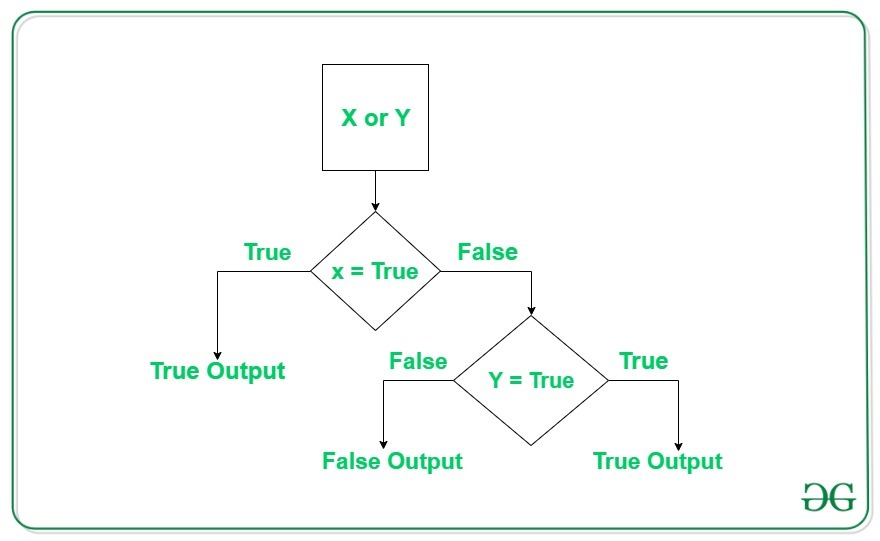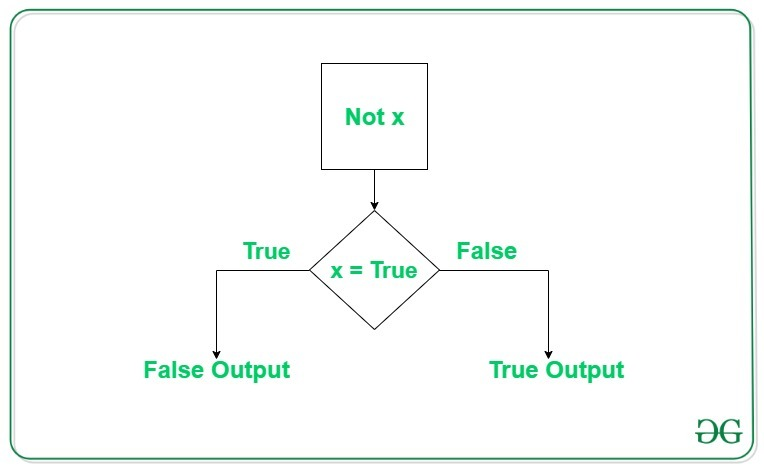# Python Logical Operators with Examples [Improvement needed]

Operators are used to perform operations on values and variables. These are the special symbols that carry out arithmetic and logical computations. The value the operator operates on is known as Operand.

Table of Content

## Logical operators

In Python, Logical operators are used on conditional statements (either True or False). They perform Logical AND, Logical OR and Logical NOT operations.

OPERATOR DESCRIPTION SYNTAX
and Logical AND: True if both the operands are true x and y
or Logical OR: True if either of the operands is true x or y
not Logical NOT: True if operand is false not x

#### Logical AND operator

Logical operator returns `True `if both the operands are True else it returns `False`.Example #1:

 `# Python program to demonstrate ` `# logical and operator ` ` `  `a ``=` `10` `b ``=` `10` `c ``=` `-``10` ` `  `if` `a > ``0` `and` `b > ``0``: ` `    ``print``(``"The numbers are greater than 0"``) ` ` `  `if` `a > ``0` `and` `b > ``0` `and` `c > ``0``: ` `    ``print``(``"The numbers are greater than 0"``) ` `else``: ` `    ``print``(``"Atleast one number is not greater than 0"``) `

Output:

```The numbers are greater than 0
Atleast one number is not greater than 0
```

Example #2:

 `# Python program to demonstrate ` `# logical and operator ` ` `  `a ``=` `10` `b ``=` `12` `c ``=` `0` ` `  `if` `a ``and` `b ``and` `c: ` `    ``print``(``"All the numbers have boolean value as True"``) ` `else``: ` `    ``print``(``"Atleast one number has boolean value as False"``) `

Output:

```Atleast one number has boolean value as False
```

Note: If the first expression evaluated to be false while using and operator, then the further expressions are not evaluated.

#### Logical or operator

Logical or operator returns True if either of the operands is True.Example #1:

 `# Python program to demonstrate ` `# logical or operator ` ` `  `a ``=` `10` `b ``=` `-``10` `c ``=` `0` ` `  `if` `a > ``0` `or` `b > ``0``: ` `    ``print``(``"Either of the number is greater than 0"``) ` `else``: ` `    ``print``(``"No number is greater than 0"``) ` ` `  `if` `b > ``0` `or` `c > ``0``: ` `    ``print``(``"Either of the number is greater than 0"``) ` `else``: ` `    ``print``(``"No number is greater than 0"``) `

Output:

```Either of the number is greater than 0
No number is greater than 0
```

Example #2:

 `# Python program to demonstrate ` `# logical and operator ` ` `  `a ``=` `10` `b ``=` `12` `c ``=` `0` ` `  `if` `a ``or` `b ``or` `c: ` `    ``print``(``"Atleast one number has boolean value as True"``) ` `else``: ` `    ``print``(``"All the numbers have boolean value as False"``) `

Output:

```Atleast one number has boolean value as True
```

Note: If the first expression evaluated to be True while using or operator, then the further expressions are not evaluated.

#### Logical not operator

Logical not operator work with the single boolean value. If the boolean value is `True `it returns `False `and vice-versa.Example:

 `# Python program to demonstrate ` `# logical not operator ` ` `  `a ``=` `10` ` `  `if` `not` `a: ` `    ``print``(``"Boolean value of a is True"``) ` ` `  `if` `not` `(a``%``3` `=``=` `0` `or` `a``%``5` `=``=` `0``): ` `    ``print``(``"10 is not divisible by either 3 or 5"``) ` `else``: ` `    ``print``(``"10 is divisible by either 3 or 5"``) `

Output:

```10 is divisible by either 3 or 5
```

## Order of evaluation of logical operators

In the case of multiple operators, Python always evaluates the expression from left to right. This can be verified by the below example.

Example:

 `# Python program to demonstrate ` `# order of evaluation of logical  ` `# operators ` ` `  `def` `order(x): ` `    ``print``(``"Method called for value:"``, x) ` `    ``return` `True` `if` `x > ``0` `else` `False` `     `  `a ``=` `order ` `b ``=` `order ` `c ``=` `order ` ` `  `if` `a(``-``1``) ``or` `b(``5``) ``or` `c(``10``): ` `    ``print``(``"Atleast one of the number is positive"``) `

Output:

```Method called for value: -1
Method called for value: 5
Atleast one of the number is positive
```

My Personal Notes arrow_drop_upCheck out this Author's contributed articles.

If you like GeeksforGeeks and would like to contribute, you can also write an article using contribute.geeksforgeeks.org or mail your article to contribute@geeksforgeeks.org. See your article appearing on the GeeksforGeeks main page and help other Geeks.

Please Improve this article if you find anything incorrect by clicking on the "Improve Article" button below.

Article Tags :

Be the First to upvote.

Please write to us at contribute@geeksforgeeks.org to report any issue with the above content.